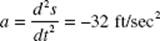﻿ ﻿BASIC DEFINITIONS - Differential Equations - Calculus AB and Calculus BC

## CHAPTER 9 Differential Equations

Concepts and Skills

In this chapter, we review how to write and solve differential equations, specifically,

• writing differential equations to model dynamic situations;

• understanding a slope field as a graphical representation of a differential equation and its solutions;

• finding general and particular solutions of separable differential equations;

• and using differential equations to analyze growth and decay.

We also review two additional BC Calculus topics:

• Euler’s method to estimate numerical solutions

• and using differential equations to analyze logistic growth and decay.

### A. BASIC DEFINITIONS

Differential equation

A differential equation (d.e.) is any equation involving a derivative. In §E of Chapter 5 we solved some simple differential equations. In Example 50, we were given the velocity at time t of a particle moving along the x-axis:From this we found the antiderivative:If the initial position (at time t = 0) of the particle is x = 3, then

x(0) = 0 − 0 + C = 3,

and C = 3. So the solution to the initial-value problem isA solution of a d.e. is any function that satisfies it. We see from (2) above that the d.e. (1) has an infinite number of solutions—one for each real value of C. We call the family of functions (2) the general solution of the d.e. (1). With the given initial condition x(0) = 3, we determined C, thus finding the unique solution (3). This is called the particular solution.

Note that the particular solution must not only satisfy the differential equation and the initial condition, but the function must also be differentiable on an interval that contains the initial point. Features such as vertical tangents or asymptotes restrict the domain of the solution. Therefore, even when they are defined by the same algebraic representation, particular solutions with different initial points may have different domains. Determining the proper domain is an important part of finding the particular solution.

In §A of Chapter 8 we solved more differential equations involving motion along a straight line. In §B we found parametric equations for the motion of a particle along a plane curve, given d.e.’s for the components of its acceleration and velocity.

Rate of Change

A differential equation contains derivatives. A derivative gives information about the rate of change of a function. For example:

(1) If P is the size of a population at time t, then we can interpret the d.e.as saying that at any time t the rate at which the population is growing is proportional (3.25%) to its size at that time.

(2) The d.e.tells us that at any time t the rate at which the quantity Q is decreasing is proportional (0.0275%) to the quantity existing at that time.

(3) In psychology, one typical stimulus-response situation, known as logarithmic response, is that in which the response y changes at a rate inversely proportional to the strength of the stimulus x. This is expressed neatly by the differential equationIf we suppose, further, that there is no response when x = x0, then we have the condition y = 0 when x = x0.

(4) We are familiar with the d.e.for the acceleration due to gravity acting on an object at a height s above ground level at time t. The acceleration is the rate of change of the object’s velocity.

﻿